# Diamond

The diamond has a circumference of 48cm. Calculate the length of its side in dm.

a =  1.2 dm

### Step-by-step explanation: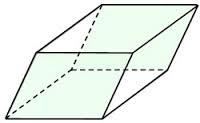Did you find an error or inaccuracy? Feel free to write us. Thank you!#### You need to know the following knowledge to solve this word math problem:

We encourage you to watch this tutorial video on this math problem:

## Related math problems and questions:

• Perimeter of the park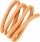Find the perimeter of the park in meters in a diamond shape, if the length of its side is 2 km.
• Triangle ABCIn a triangle ABC side b measure 10 cm less than the side a and side b is half of the side c. Calculate the length of sides if the circumference of the triangle is 42 cm.
• RhombusFind the length of each side of rhombus if the perimeter is 89 cm long.
• Table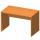Find the circumference of the table, where the long side is 1.28 meters and the short side is 86 cm.
• RectangleThe length of one side of the rectangle is three times the length of the second side. What are the dimensions of the rectangle if its circumference 96 cm?
• Diamond - simple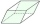Calculate the circumference and area of the diamond if the side is 5 cm long and the height is 3 cm long.
• Strip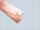From 5.9 cm wide strip should be cut rhombus with area 28 cm2. How long will be its side?
• Calculate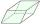Calculate the content cm2 diamond with side length 9.8 cm and a height on the side of 5 cm.
• The diagonals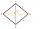The diagonals of the KOSA diamond are 20 cm and 48 cm long. Calculate the circumference of the diamond in centimeters rounded to one unit.
• Second sideCalculate the length of the other side of the rectangle if its circumference is 60 cm and one side is 10 cm long.
• Fence of the garden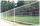The garden has dimensions of 5m and 400cm. How many meters meshes are needed for fencing the plot?
• Christmas napkinsThe girls embroidered Christmas napkins. Each napkin had the shape of a triangle with sides of 5 dm, 60 cm, and 800 mm. How many cms did the girls sew if they made 15 napkins?
• Isosceles triangle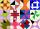Calculate the perimeter of isosceles triangle with arm length 73 cm and base length of 48 cm.
• Perimeter of rhombus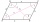The area of the rhombus is 32 cm2. One side of it measures 10 cm. The height on the other side measures 5 cm. What is the circumference of this rhombus?
• Rhombus sidesfind the sides of a rhombus, it area is 550 cm square and altitude is 55cm
• RectanglesHow many different rectangles with sides integers (in mm) have a circumference exactly 1000 cm? (a rectangle with sides of 50cm and 450cm is considered to be the same as a rectangle with sides of 450cm and 50cm)
• The parallelogram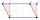The parallelogram has one side 2 dm long, which is one-sixth of its circumference. How many cm does the other side of the parallelogram measure?